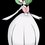# Solving Pell Equation of Norms other than -1 and 1

People, I crossed a Pell-type equation: x^2 - 6y^2 = 3 which it has norm 3. Are there ways to solve this equation without using concepts from Abstract Algebra such as factoring in a number field or what?Note by John Ashley Capellan
4 years, 7 months ago

MarkdownAppears as
*italics* or _italics_ italics
**bold** or __bold__ bold
- bulleted- list
• bulleted
• list
1. numbered2. list
1. numbered
2. list
Note: you must add a full line of space before and after lists for them to show up correctly
paragraph 1paragraph 2

paragraph 1

paragraph 2

[example link](https://brilliant.org)example link
> This is a quote
This is a quote
    # I indented these lines
# 4 spaces, and now they show
# up as a code block.

print "hello world"
# I indented these lines
# 4 spaces, and now they show
# up as a code block.

print "hello world"
MathAppears as
Remember to wrap math in $$...$$ or $...$ to ensure proper formatting.
2 \times 3 $$2 \times 3$$
2^{34} $$2^{34}$$
a_{i-1} $$a_{i-1}$$
\frac{2}{3} $$\frac{2}{3}$$
\sqrt{2} $$\sqrt{2}$$
\sum_{i=1}^3 $$\sum_{i=1}^3$$
\sin \theta $$\sin \theta$$
\boxed{123} $$\boxed{123}$$

Sort by:

@John Ashley Capellan Can you add what you learnt about Pell's Equation to the Wiki? Thanks!

Staff - 4 years, 5 months ago

You can read up on Pell's Equation, to understand how to generate solutions from a base case.

1) Observe that $$(5,2)$$ is the first non-trivial solution to $$x^2 - 6y^2 = 1$$.

2) Observe that

$( a^2 - 6b^2 ) ( c^2 - 6 d^2 ) = a^2c^2 + 36 b^2d^2 - 6 b^2 c^2 - 6a^2d^2= ( ac + 6bd) ^2 - 6 ( bc+ad) ^2.$

As such, we define pair-multiplication as $$(a,b) \otimes (c, d) = ( ac + 6 bd , bc + ad)$$.

3) Observe that $$(3, 1)$$ is a solution to $$x^2 - 6y^2 = 3$$.

4) Hence, solutions exist in the form of $$(3,1) \otimes ( 5,2)^ n$$, where $$n$$ is a non-negative integer.
For example, with $$n=1$$, we get $$(3,1) \otimes (5,2) = ( 15 + 12 , 5 + 6) = (27, 11)$$. We can check that $$27^2 - 6 \times 11^2 = 729 - 726 = 3$$.

Followup question: Are there other solutions? (ignore negative values)

Staff - 4 years, 7 months ago

You also left out an $$a^2$$ in Point 2. Cheers, G.

- 7 months, 1 week ago

Fixed. Thanks!

Staff - 7 months, 1 week ago

It's $$(ac+6bd,bc+ad)$$. Fix it to avoid confusion.

- 4 years, 2 months ago

First find the smallest positive solution of x,y. Express it as x+6^0.5y Then find solution of the equation x^2-6y^2=1. Express it as x+6^0.5y Multiply any of these two you get another solution. Hence obtain all solutions.

- 4 years, 7 months ago

Could you please explain in a bit more detail? Sorry for the trouble.

- 4 years, 7 months ago

You can know about my solution by searching pell fermat equation in wikipedia

- 4 years, 7 months ago